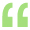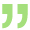PCC - Tax - House Property - Pre-construction period Query

Page no : 2

Replied 10 March 2010

CA Birendra Gupta (Looking for job) (43 Points)
Replied 10 March 2010

 Originally posted by : CA PCC StudentHello Friends,   I have a small query in PCC Taxation, in the topic Income from House Property, Pre-Construction Period Calculation. Here is the question in my book :   B purchased a piece of land, the built-up area of house was 1,000 sq ft. Ground Floor and an equal area in the first floor. B Started the construction on 1.4.2008 and completed the construction on 1.7.2009. B Occupied the ground floor on 1.7.2009 and let-out the First Floor for a rent of Rs.15,000 p.m. However, the tenant vacated the house on 31.12.2009 and B occupied the entire house during the period 1.1.2010 to 31.3.2010. B has availed a housing loan of Rs.12 Lakhs at 10% p.a on 1.7.2008. No repayment was made by him till 31.3.2010. So in this question i've calculated current period interest as follows : For the purpose of Self Occupied and Let out portion, i'm taking 50%. 12,00,000*10%*50% = 60,000   This is what I did but in my text book padhuka its given in this way which i didn't understand :   current period interest  ------- 12,00,000*10%*50% = 60,000 prior period interest ----------- 12,00,000*10%*9/12*50%*1/5 = 9,000 I understood why we have taken 1/5 but i didn't understand why 9/12 is considered.   Please explain me pre-construction period in this case fully in detail.   Thank you..!! Here pre const. period interest is taken from the date from when the loan is taken i.e from 1.7.2008 to 31.3.2009 not the date on which the const started i.e 1.04.2008, date on which loan is taken is relevent for computation of pre const period int.V CHANDRA SEKARA RAJU (CA FINAL) (908 Points)
Replied 10 March 2010

Before going to the problem we have to know what is the Preconstruction Period.

Preconstruction Period means, it is the period not form part of the previous year and starts from the date on which loan was taken up and ends with year ending, which is immediatly preceeding to the previous year..

For Example..:

Construction  a house is started on 01.05.2006 & and loan taken for Construction is on 01.07.2007 and subsequently house is completed on 01.07.2009.......then Preconstruction Period starts from 01.07.2007 and ends with 31.03.2009.....

in your question Preconstruction period starts from 01.07.2008 and ends with 31.03.2009

so that calculation of interest is of 9months only...

i.e;

• 12,00,000*10/100*9/12*50% =  45000

It is allowed as deduction U/s 24 for 5 succeding precious years in equal instalments.....

so that deduction for AY 2010-11 is 45000/5 = 9000

and current year interest is 12,00,000*10/100*50% = 60000

Hence total deduction U/s 24 is 69000/-

CA Birendra Gupta (Looking for job) (43 Points)
Replied 10 March 2010

Here pre const. period interest is taken from the date from when the loan is taken i.e from 1.7.2008 to 31.3.2009 not the date on which the const started i.e 1.04.2008, date on which loan is taken is relevent for computation of pre const period int.

CA Birendra Gupta (Looking for job) (43 Points)
Replied 10 March 2010

 Originally posted by : CA PCC StudentHello Friends,   I have a small query in PCC Taxation, in the topic Income from House Property, Pre-Construction Period Calculation. Here is the question in my book :   B purchased a piece of land, the built-up area of house was 1,000 sq ft. Ground Floor and an equal area in the first floor. B Started the construction on 1.4.2008 and completed the construction on 1.7.2009. B Occupied the ground floor on 1.7.2009 and let-out the First Floor for a rent of Rs.15,000 p.m. However, the tenant vacated the house on 31.12.2009 and B occupied the entire house during the period 1.1.2010 to 31.3.2010. B has availed a housing loan of Rs.12 Lakhs at 10% p.a on 1.7.2008. No repayment was made by him till 31.3.2010. So in this question i've calculated current period interest as follows : For the purpose of Self Occupied and Let out portion, i'm taking 50%. 12,00,000*10%*50% = 60,000   This is what I did but in my text book padhuka its given in this way which i didn't understand :   current period interest  ------- 12,00,000*10%*50% = 60,000 prior period interest ----------- 12,00,000*10%*9/12*50%*1/5 = 9,000 I understood why we have taken 1/5 but i didn't understand why 9/12 is considered.   Please explain me pre-construction period in this case fully in detail.   Thank you..!!Here pre const. period interest is taken from the date from when the loan is taken i.e from 1.7.2008 to 31.3.2009 not the date on which the const started i.e 1.04.2008, date on which loan is taken is relevent for computation of pre const period int.

Prasanth Nair V (ACA,ACS,CWA Final,Mcom) (557 Points)
Replied 10 March 2010

hi.. The Interest comes around 120000(12 lacs @ 10%) for an entire year. but in this case he has availed the loan for only 9 months. so only 9/12 of 120000 equal to 90000.

This is for the whole house. for the let out part,it comes to 45000(half of 90000).

this is allowed in 5 equal instalments

thus 1200000*10%*9/12*50%*1/5.. \

Hope u Understood..view more »

expand_less ﻿